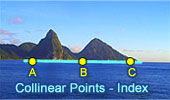# Collinear Points, Theorems and Problems - Table of Content 9Proposed Problem 323.
Triangle, Incenter, Circumcenter, Equal circles, Collinearity.

Proposed Problem 321.
Triangle, Incenter, Excircle, Midpoint of the Altitude, Collinearity.

Proposed Problem 320.
Triangle, Circumcircle, Incenter, Excenter, Collinear points.

Proposed Problem 318.
A Scalene triangle, Altitudes, Collinear points.

Proposed Problem 319.
Triangle, Altitudes, Perpendiculars, Collinear points.

Go to page:  Previous | 1 | 2 | 3 | 4 | 5 | 6 | 7 | 8 | 9 | 10 | Next

Home | SearchGeometry | Lines | Post a comment | Email | By Antonio Gutierrez